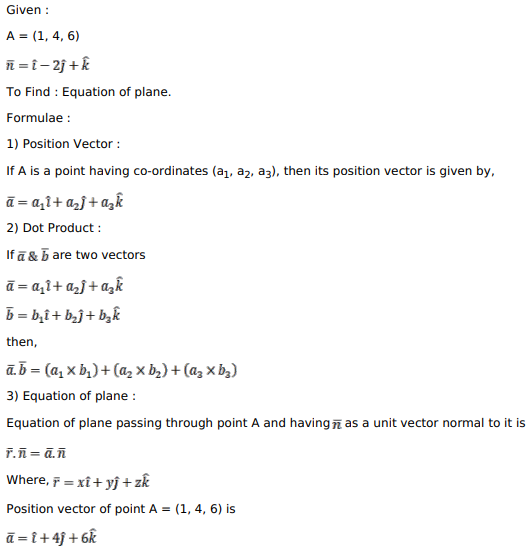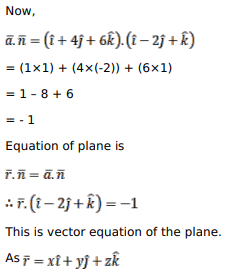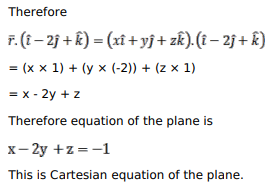# Find the vector, and Cartesian equations of a plane which passes through the pointQuestion:

Find the vector, and Cartesian equations of a plane which passes through the point $(1,4,6)$ and the normal vector to the plane is $(\hat{i}-2 \hat{j}+\hat{k})$.

Solution: